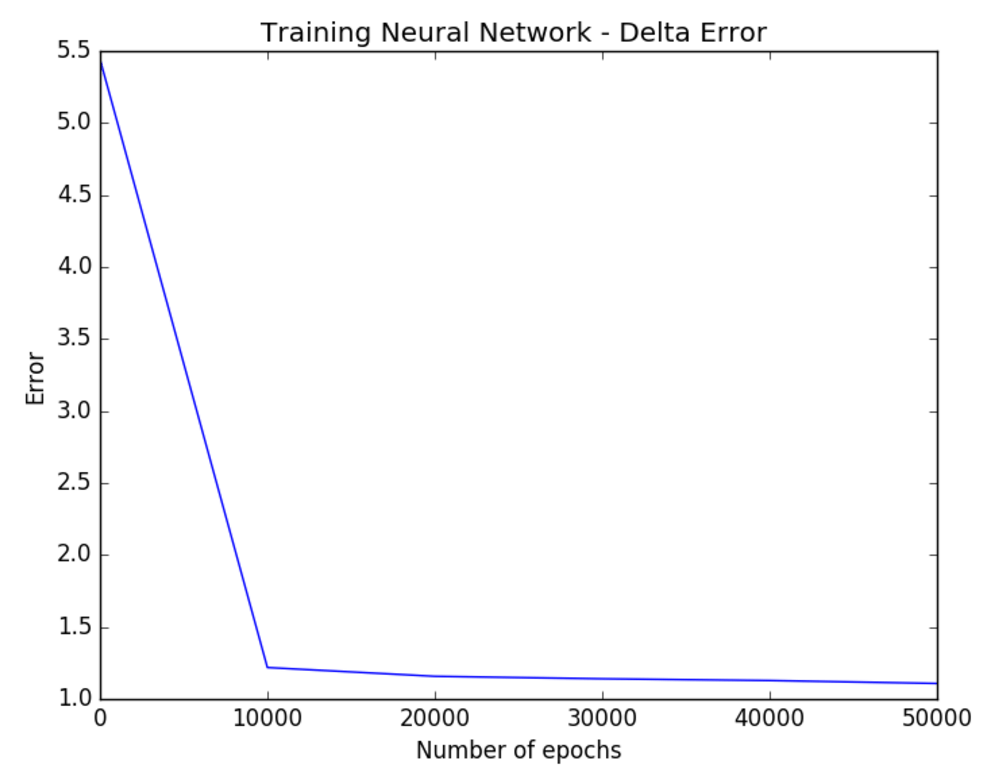# Iris Plant Classification Using Neural Network – Online Experiments with Normalization and Other Parameters

Do we need to normalize input data for neural network? How differently will be results from running normalized and non normalized data? This will be explored in the post using Online Machine Learning Algorithms tool for classification of iris data set with feed-forward neural network.

Feed-forward Neural Network
Feed-forward neural networks are commonly used for classification. In this example we choose feed-forward neural network with back propagation training and gradient descent optimization method.

Our neural network has one hidden layer. The number of neuron units in this hidden layer and learning rate can be set by user. We do not need to code neural network because we use online tool that is taking training, testing data, number of neurons in hidden layer and learning rate parameters as input.

The output from this tool is classification results for testing data set, neural network weights and deltas at different iterations.

Data Set
As mentioned above we use iris data set for data input to neural network. The data set contains 3 classes of 50 instances each, where each class refers to a type of iris plant.  We remove first 3 rows for each class and save for testing data set. Thus the input data set has 141 rows and testing data set has 9 rows.

Normalization
We run neural network first time without normalization of input data. Then we run neural network with normalized data.
We use min max normalization which can be described by formula :
xij = (xij – colmmin) /(colmmax – colmmin)

Where
colmmin = minimum value of the column
colmmax = maximum value of the column
xij = x(data item) present at ithrow and jthcolumn

Normalization is applied to both X and Y, so the possible values for Y become 0, 05, 1
and X become like :
0.083333333 0.458333333 0.084745763 0.041666667

You can view normalized data at this link : Iris Data Set – Normalized Data

Online Tool
Once data is ready we can to load data to neural network and run classification. Here are the steps how to use online Machine Learning Algorithms tool:
1.Access the link Online Machine Learning Algorithms with feed-forward neural network.
2.Select algorithm and click Load parameters for this model.
3.Input the data that you want to run.
4.Click Run now.
6.Click Refresh button on this new page, you maybe will need click few times untill you see data output.
7.Scroll to the bottom page to see calculations.
For more detailed instruction use this link: How to Run Online Machine Learning Algorithms Tool

Experiments
We do 4 experiments as shown in the table below: 1 without normalization, and 3 with normalization and various learning rate and number of neurons hidden. Results are shown in the same table and the error graph shown below under the table. When iris data set was inputted without normalization, the text label is changed to numerical variable with values -1,0,1 The result is not satisfying with needed accuracy level. So decision is made to normalize data using min max method.

Here is the sample result from the last experiment, with the lowest error. The learning rate is 0.1 and the number of hidden neurons is 36. The delta error in the end of training is 1.09.

[[ 0.00416852]
[ 0.01650389]
[ 0.01021347]
[ 0.43996485]
[ 0.50235484]
[ 0.58338683]
[ 0.80222148]
[ 0.92640374]
[ 0.93291573]]

Results

 Normalization Learn. Rate Hidden units # Correct Total Accuracy Delta Error No 0.5 4 3 9 33% 9.7 Yes (Min Max) 0.5 4 8 9 89% 1.25 Yes (Min Max) 0.1 4 9 9 100% 1.19 Yes (Min Max) 0.1 36 9 9 100% 1.09Conclusion
Normalization can make huge difference on results. Further improvements are posible by adjusting learning rate or number hidden units in the hidden layer.

References